# Algorithm Analysis and Recursion 1 Algorithm An algorithm

• Slides: 56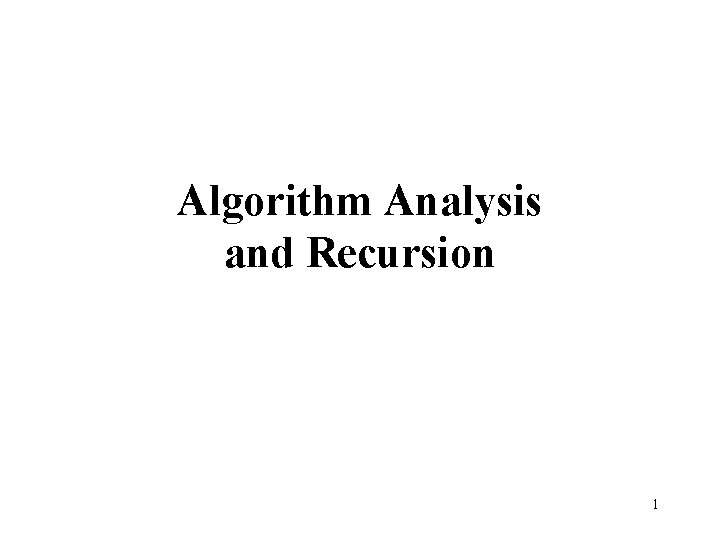Algorithm Analysis and Recursion 1Algorithm • An algorithm is a set of instructions to be followed to solve a problem. – There can be more than one solution (more than one algorithm) to solve a given problem. – An algorithm can be implemented using different programming languages on different platforms. • An algorithm must be correct. It should correctly solve the problem. – e. g. For sorting, this means even if (1) the input is already sorted, or (2) it contains repeated elements. • Once we have a correct algorithm for a problem, we have to determine the efficiency of that algorithm. 2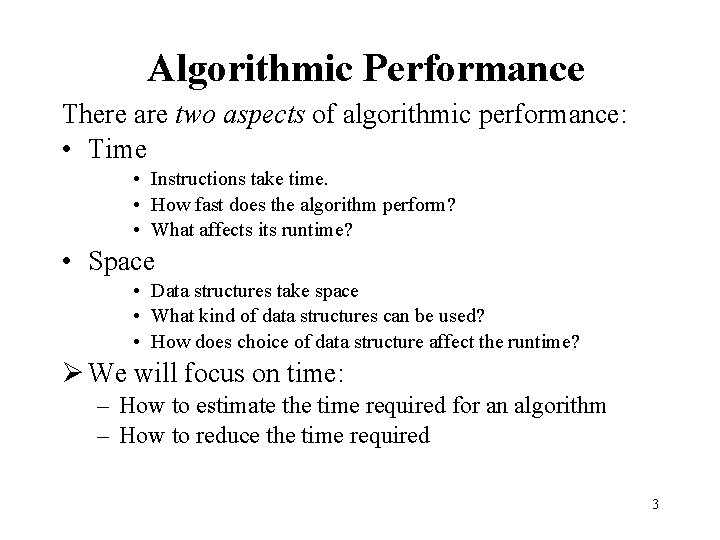Algorithmic Performance There are two aspects of algorithmic performance: • Time • Instructions take time. • How fast does the algorithm perform? • What affects its runtime? • Space • Data structures take space • What kind of data structures can be used? • How does choice of data structure affect the runtime? We will focus on time: – How to estimate the time required for an algorithm – How to reduce the time required 3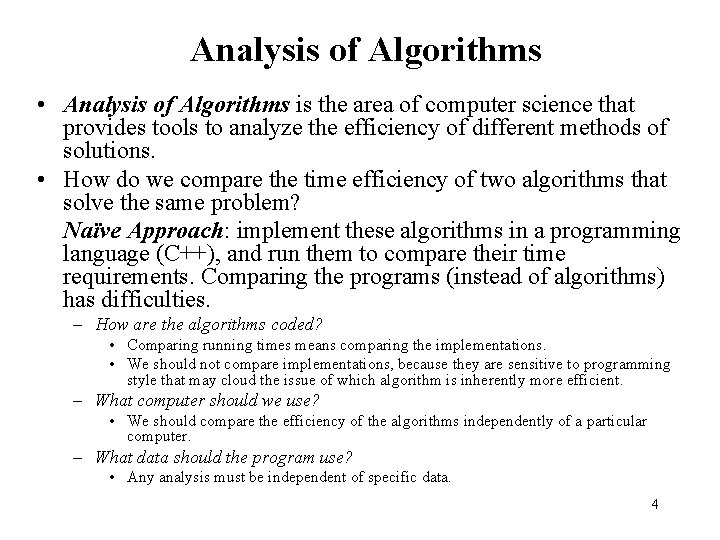Analysis of Algorithms • Analysis of Algorithms is the area of computer science that provides tools to analyze the efficiency of different methods of solutions. • How do we compare the time efficiency of two algorithms that solve the same problem? Naïve Approach: implement these algorithms in a programming language (C++), and run them to compare their time requirements. Comparing the programs (instead of algorithms) has difficulties. – How are the algorithms coded? • Comparing running times means comparing the implementations. • We should not compare implementations, because they are sensitive to programming style that may cloud the issue of which algorithm is inherently more efficient. – What computer should we use? • We should compare the efficiency of the algorithms independently of a particular computer. – What data should the program use? • Any analysis must be independent of specific data. 4Analysis of Algorithms • When we analyze algorithms, we should employ mathematical techniques that analyze algorithms independently of specific implementations, computers, or data. • To analyze algorithms: – First, we start to count the number of significant operations in a particular solution to assess its efficiency. – Then, we will express the efficiency of algorithms using growth functions. 5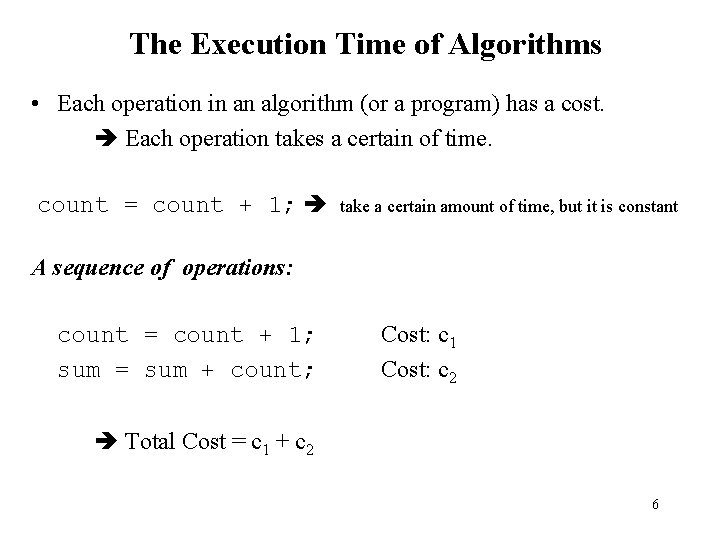The Execution Time of Algorithms • Each operation in an algorithm (or a program) has a cost. Each operation takes a certain of time. count = count + 1; take a certain amount of time, but it is constant A sequence of operations: count = count + 1; sum = sum + count; Cost: c 1 Cost: c 2 Total Cost = c 1 + c 2 6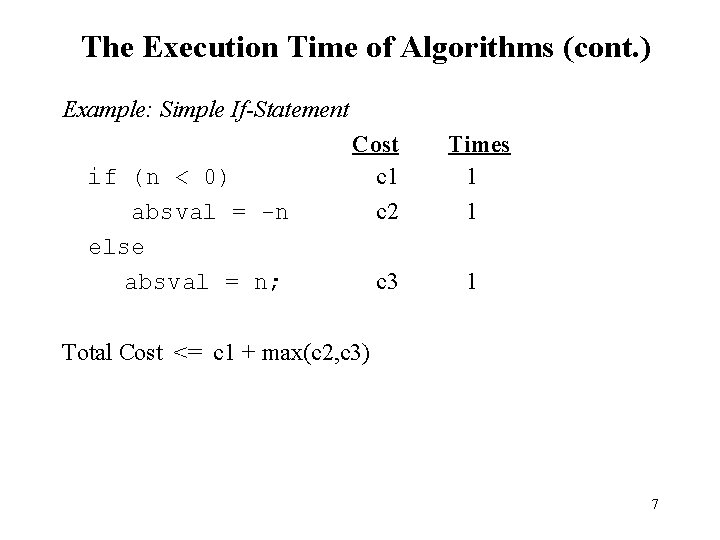The Execution Time of Algorithms (cont. ) Example: Simple If-Statement Cost if (n < 0) c 1 absval = -n c 2 else absval = n; c 3 Times 1 1 1 Total Cost <= c 1 + max(c 2, c 3) 7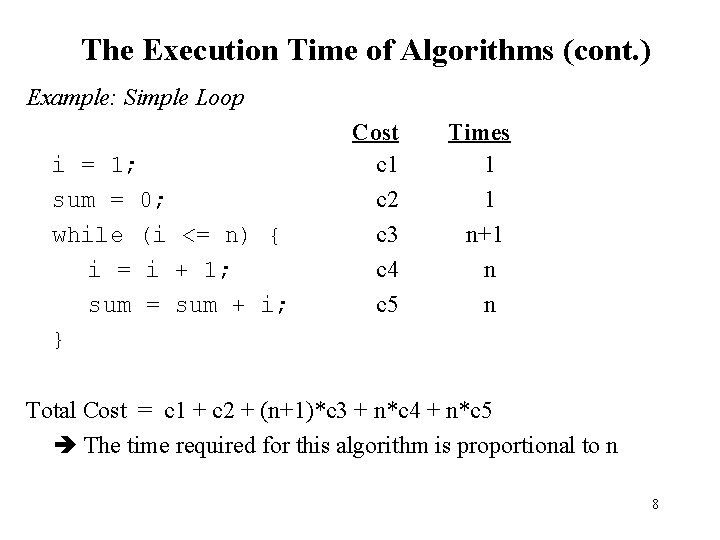The Execution Time of Algorithms (cont. ) Example: Simple Loop i = 1; sum = 0; while (i <= n) { i = i + 1; sum = sum + i; } Cost c 1 c 2 c 3 c 4 c 5 Times 1 1 n+1 n n Total Cost = c 1 + c 2 + (n+1)*c 3 + n*c 4 + n*c 5 The time required for this algorithm is proportional to n 8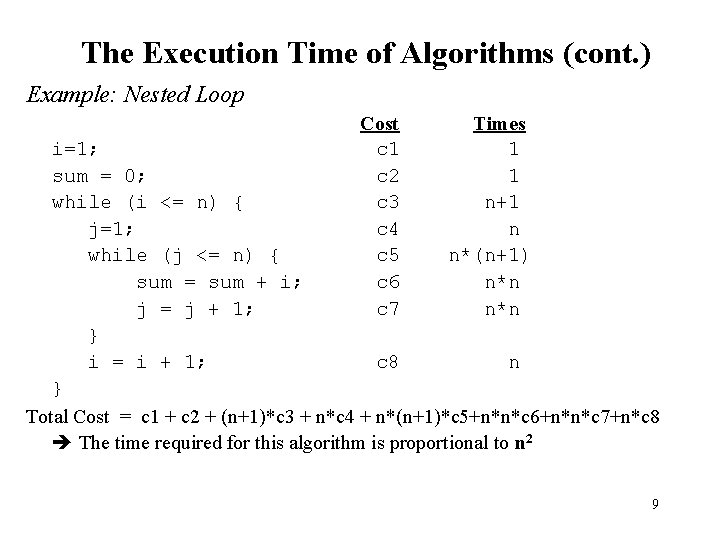The Execution Time of Algorithms (cont. ) Example: Nested Loop Cost c 1 c 2 c 3 c 4 c 5 c 6 c 7 Times 1 1 n+1 n n*(n+1) n*n i=1; sum = 0; while (i <= n) { j=1; while (j <= n) { sum = sum + i; j = j + 1; } i = i + 1; c 8 n } Total Cost = c 1 + c 2 + (n+1)*c 3 + n*c 4 + n*(n+1)*c 5+n*n*c 6+n*n*c 7+n*c 8 The time required for this algorithm is proportional to n 2 9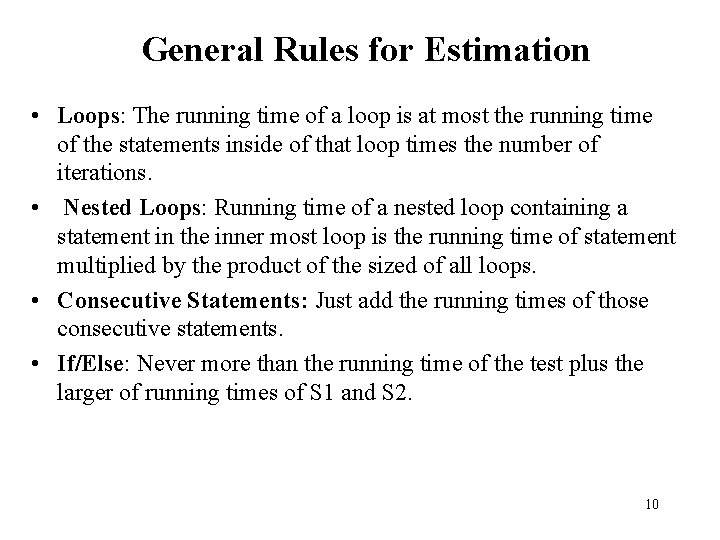General Rules for Estimation • Loops: The running time of a loop is at most the running time of the statements inside of that loop times the number of iterations. • Nested Loops: Running time of a nested loop containing a statement in the inner most loop is the running time of statement multiplied by the product of the sized of all loops. • Consecutive Statements: Just add the running times of those consecutive statements. • If/Else: Never more than the running time of the test plus the larger of running times of S 1 and S 2. 10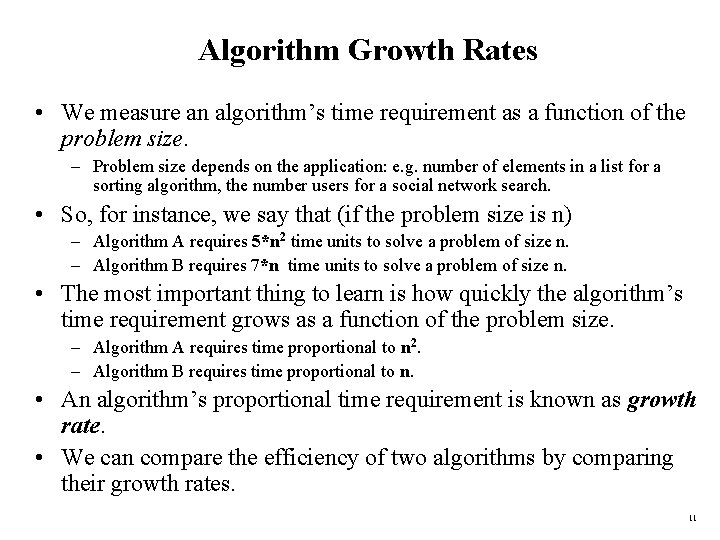Algorithm Growth Rates • We measure an algorithm’s time requirement as a function of the problem size. – Problem size depends on the application: e. g. number of elements in a list for a sorting algorithm, the number users for a social network search. • So, for instance, we say that (if the problem size is n) – Algorithm A requires 5*n 2 time units to solve a problem of size n. – Algorithm B requires 7*n time units to solve a problem of size n. • The most important thing to learn is how quickly the algorithm’s time requirement grows as a function of the problem size. – Algorithm A requires time proportional to n 2. – Algorithm B requires time proportional to n. • An algorithm’s proportional time requirement is known as growth rate. • We can compare the efficiency of two algorithms by comparing their growth rates. 11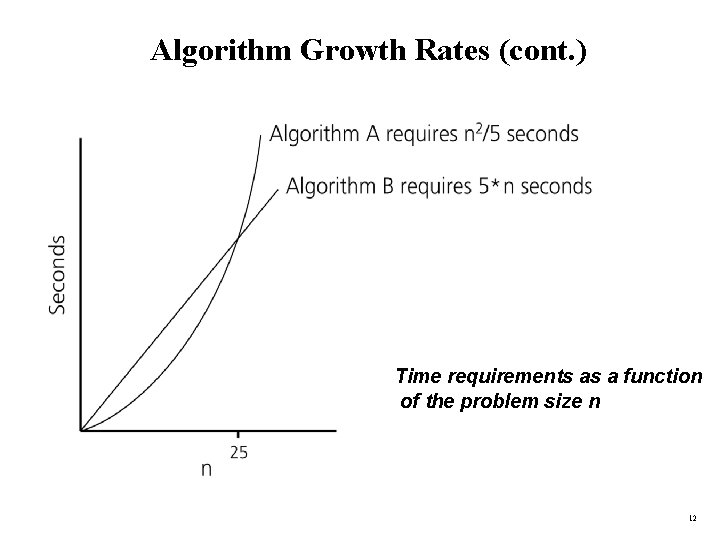Algorithm Growth Rates (cont. ) Time requirements as a function of the problem size n 12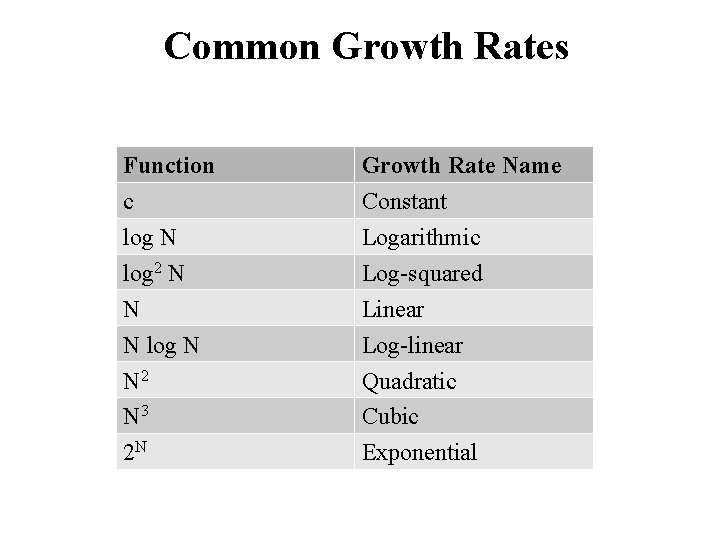Common Growth Rates Function c log N log 2 N N N log N N 2 N 3 2 N Growth Rate Name Constant Logarithmic Log-squared Linear Log-linear Quadratic Cubic Exponential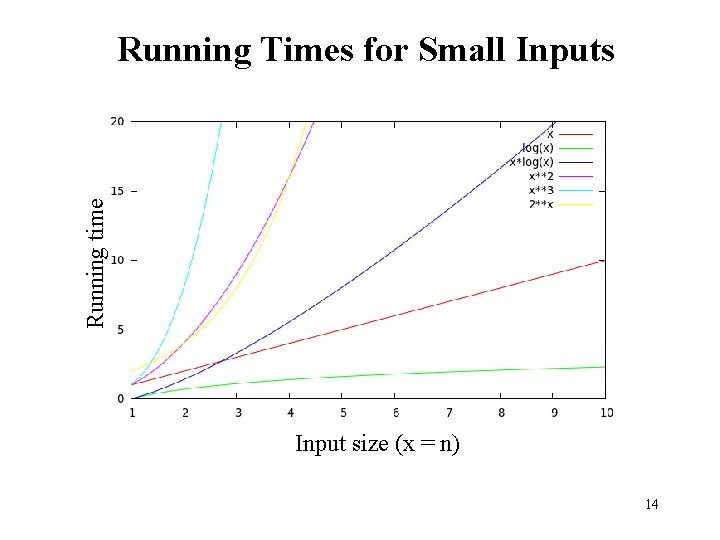Running time Running Times for Small Inputs Input size (x = n) 14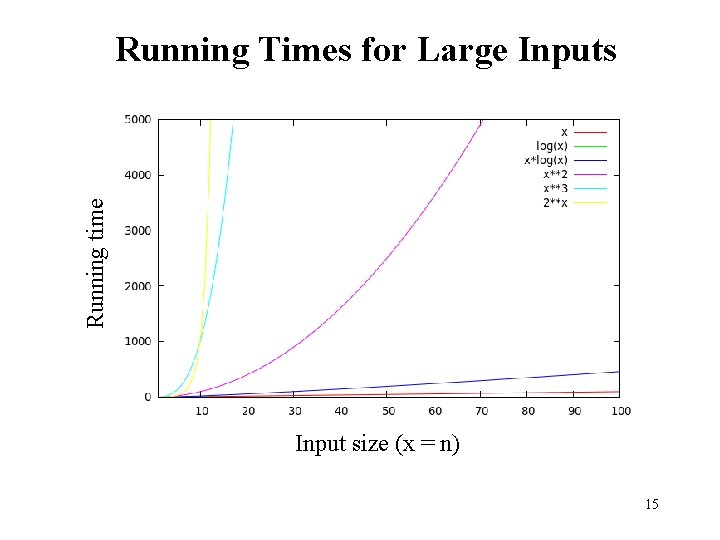Running time Running Times for Large Inputs Input size (x = n) 15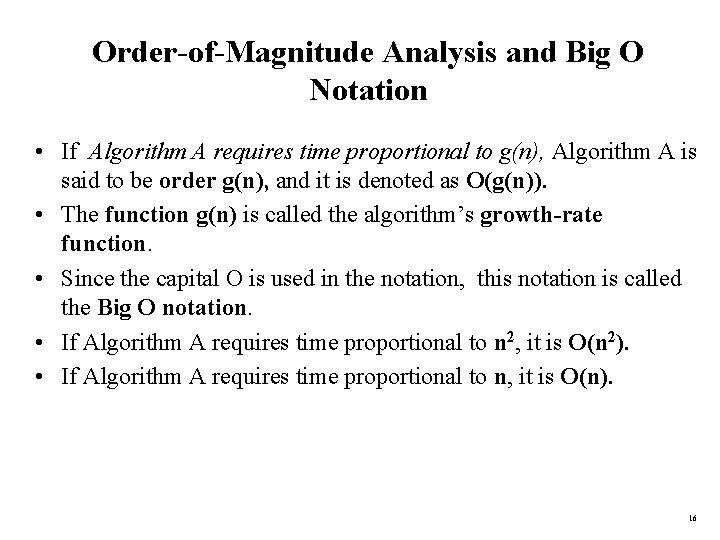Order-of-Magnitude Analysis and Big O Notation • If Algorithm A requires time proportional to g(n), Algorithm A is said to be order g(n), and it is denoted as O(g(n)). • The function g(n) is called the algorithm’s growth-rate function. • Since the capital O is used in the notation, this notation is called the Big O notation. • If Algorithm A requires time proportional to n 2, it is O(n 2). • If Algorithm A requires time proportional to n, it is O(n). 16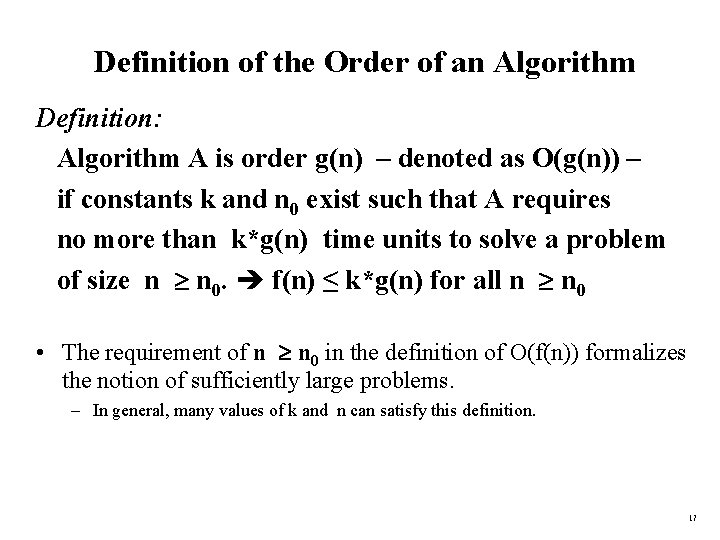Definition of the Order of an Algorithm Definition: Algorithm A is order g(n) – denoted as O(g(n)) – if constants k and n 0 exist such that A requires no more than k*g(n) time units to solve a problem of size n n 0. f(n) ≤ k*g(n) for all n n 0 • The requirement of n n 0 in the definition of O(f(n)) formalizes the notion of sufficiently large problems. – In general, many values of k and n can satisfy this definition. 17Order of an Algorithm • If an algorithm requires f(n) = n 2– 3*n+10 seconds to solve a problem size n. If constants k and n 0 exist such that k*n 2 > n 2– 3*n+10 for all n n 0. the algorithm is order n 2 (In fact, k is 3 and n 0 is 2) 3*n 2 > n 2– 3*n+10 for all n 2. Thus, the algorithm requires no more than k*n 2 time units for n n 0 , So it is O(n 2) 18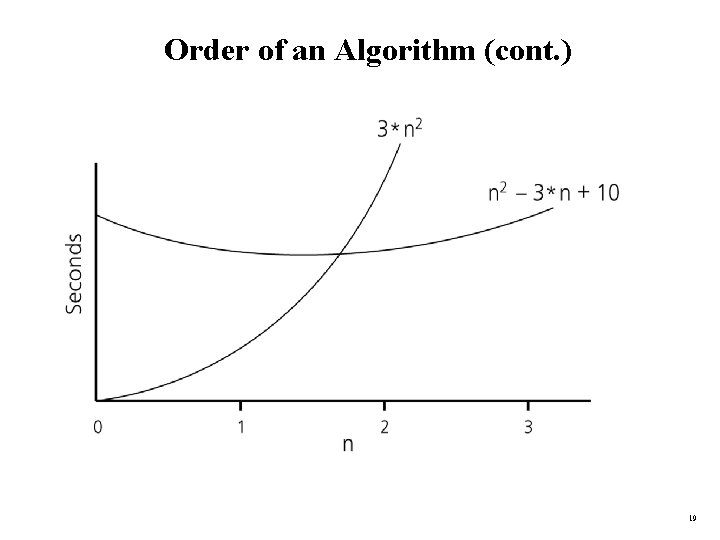Order of an Algorithm (cont. ) 19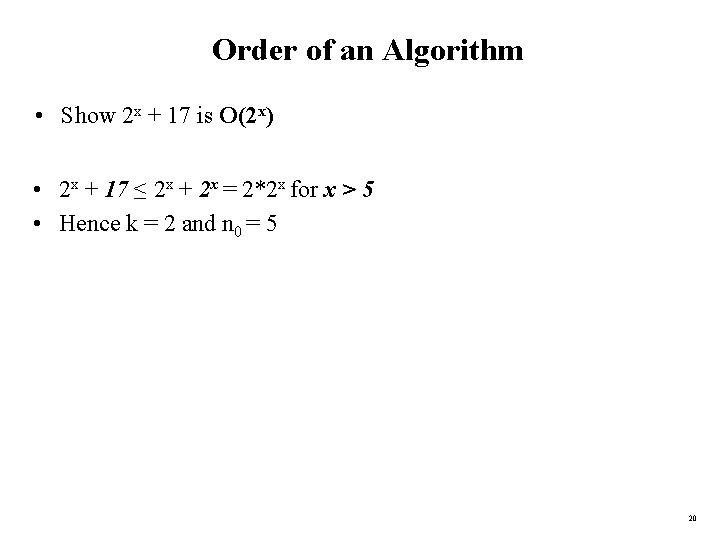Order of an Algorithm • Show 2 x + 17 is O(2 x) • 2 x + 17 ≤ 2 x + 2 x = 2*2 x for x > 5 • Hence k = 2 and n 0 = 5 20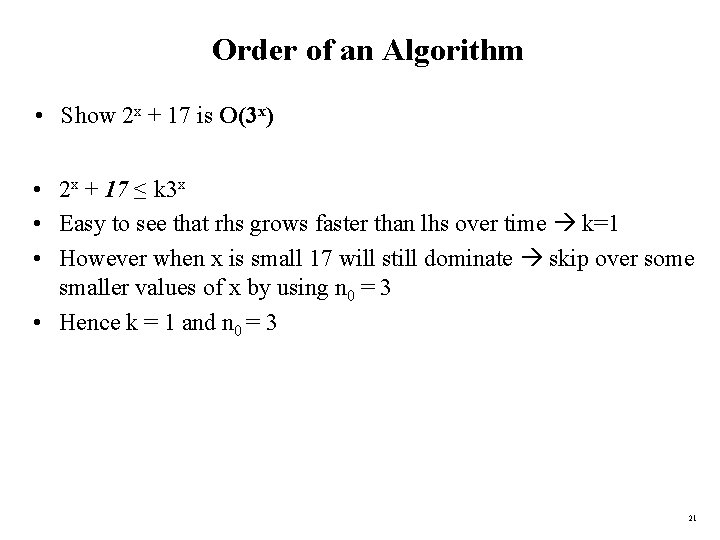Order of an Algorithm • Show 2 x + 17 is O(3 x) • 2 x + 17 ≤ k 3 x • Easy to see that rhs grows faster than lhs over time k=1 • However when x is small 17 will still dominate skip over some smaller values of x by using n 0 = 3 • Hence k = 1 and n 0 = 3 21Definition of the Order of an Algorithm Definition: Algorithm A is omega g(n) – denoted as Ω(g(n)) – if constants k and n 0 exist such that A requires more than k*g(n) time units to solve a problem of size n n 0. f(n) ≥ k*g(n) for all n n 0 22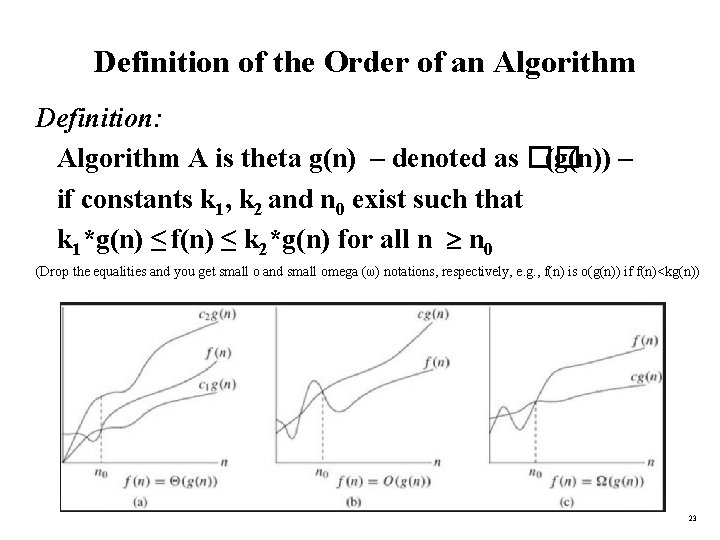Definition of the Order of an Algorithm Definition: Algorithm A is theta g(n) – denoted as �� (g(n)) – if constants k 1, k 2 and n 0 exist such that k 1*g(n) ≤ f(n) ≤ k 2*g(n) for all n n 0 (Drop the equalities and you get small o and small omega (ω) notations, respectively, e. g. , f(n) is o(g(n)) if f(n)<kg(n)) 23Order of an Algorithm • Show f(n) = 7 n 2 + 1 is �� (n 2) • • You need to show f(n) is O(n 2) and f(n) is Ω(n 2) f(n) is O(n 2) because 7 n 2 + 1 ≤ 7 n 2 + n 2 ∀n ≥ 1 k 1 = 8 n 0 = 1 f(n) is Ω (n 2) because 7 n 2 + 1 ≥ 7 n 2 ∀n ≥ 0 k 2 = 7 n 0 = 0 Pick the largest n 0 to satisfy both conditions naturally k 1 = 8, k 2 = 7, n 0 = 1 24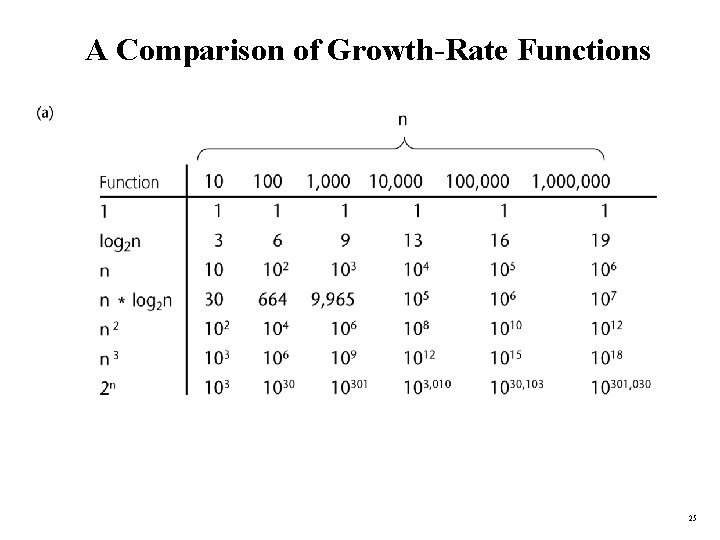A Comparison of Growth-Rate Functions 25Growth-Rate Functions O(1) Time requirement is constant, and it is independent of the problem’s size. O(log 2 n) Time requirement for a logarithmic algorithm increases slowly as the problem size increases. O(n) Time requirement for a linear algorithm increases directly with the size of the problem. O(n*log 2 n) Time requirement for a n*log 2 n algorithm increases more rapidly than a linear algorithm. O(n 2) Time requirement for a quadratic algorithm increases rapidly with the size of the problem. O(n 3) Time requirement for a cubic algorithm increases more rapidly with the size of the problem than the time requirement for a quadratic algorithm. O(2 n) As the size of the problem increases, the time requirement for an exponential algorithm increases too rapidly to be practical. 26Growth-Rate Functions • If an algorithm takes 1 second to run with the problem size 8, what is the time requirement (approximately) for that algorithm with the problem size 16? • If its order is: O(1) T(n) = 1 second O(log 2 n) T(n) = (1*log 216) / log 28 = 4/3 seconds O(n) T(n) = (1*16) / 8 = 2 seconds O(n*log 2 n) T(n) = (1*16*log 216) / 8*log 28 = 8/3 seconds O(n 2) T(n) = (1*162) / 82 = 4 seconds O(n 3) T(n) = (1*163) / 83 = 8 seconds O(2 n) T(n) = (1*216) / 28 = 28 seconds = 256 seconds 27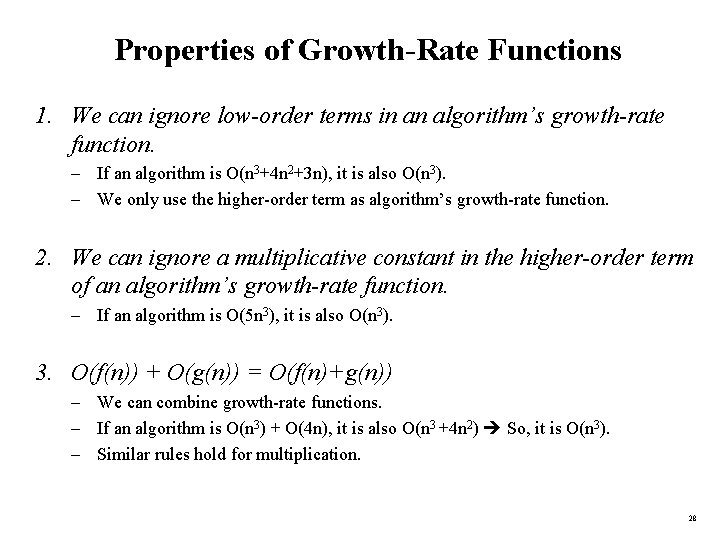Properties of Growth-Rate Functions 1. We can ignore low-order terms in an algorithm’s growth-rate function. – If an algorithm is O(n 3+4 n 2+3 n), it is also O(n 3). – We only use the higher-order term as algorithm’s growth-rate function. 2. We can ignore a multiplicative constant in the higher-order term of an algorithm’s growth-rate function. – If an algorithm is O(5 n 3), it is also O(n 3). 3. O(f(n)) + O(g(n)) = O(f(n)+g(n)) – We can combine growth-rate functions. – If an algorithm is O(n 3) + O(4 n), it is also O(n 3 +4 n 2) So, it is O(n 3). – Similar rules hold for multiplication. 28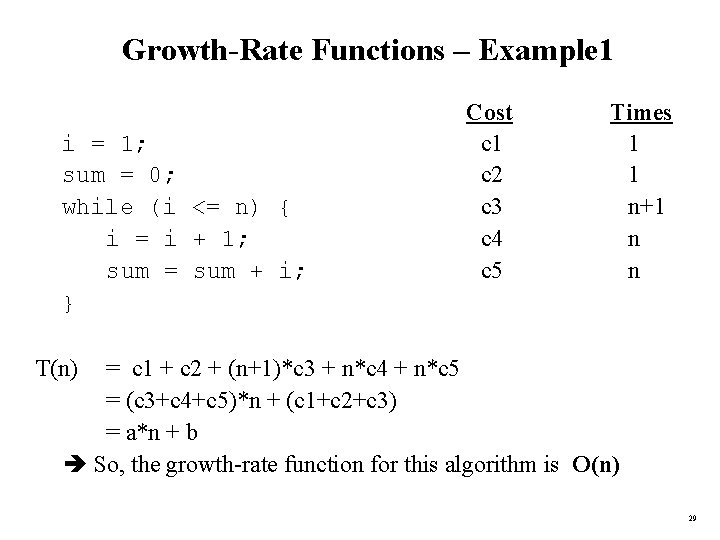Growth-Rate Functions – Example 1 i = 1; sum = 0; while (i <= n) { i = i + 1; sum = sum + i; } Cost c 1 c 2 c 3 c 4 c 5 Times 1 1 n+1 n n T(n) = c 1 + c 2 + (n+1)*c 3 + n*c 4 + n*c 5 = (c 3+c 4+c 5)*n + (c 1+c 2+c 3) = a*n + b So, the growth-rate function for this algorithm is O(n) 29Growth-Rate Functions – Example 3 for (i=1; i<=n; i++) for (j=1; j<=i; j++) for (k=1; k<=j; k++) x=x+1; T(n) = So, the growth-rate function for this algorithm is O(n 3) 30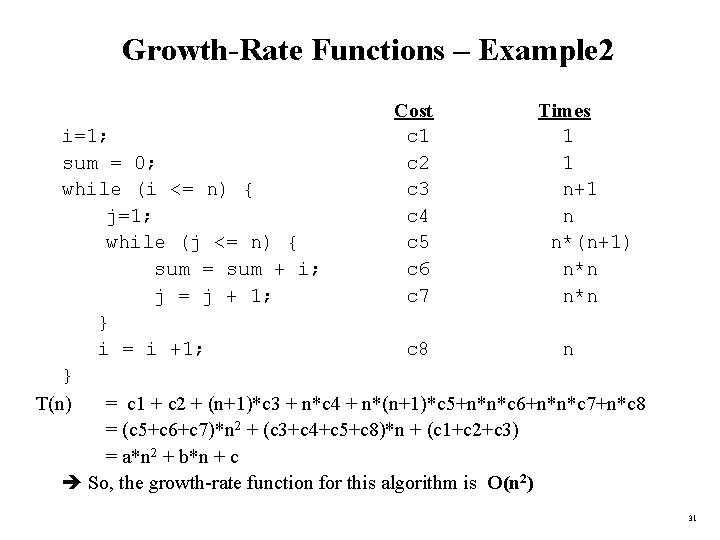Growth-Rate Functions – Example 2 Cost c 1 c 2 c 3 c 4 c 5 c 6 c 7 Times 1 1 n+1 n n*(n+1) n*n i=1; sum = 0; while (i <= n) { j=1; while (j <= n) { sum = sum + i; j = j + 1; } i = i +1; c 8 n } T(n) = c 1 + c 2 + (n+1)*c 3 + n*c 4 + n*(n+1)*c 5+n*n*c 6+n*n*c 7+n*c 8 = (c 5+c 6+c 7)*n 2 + (c 3+c 4+c 5+c 8)*n + (c 1+c 2+c 3) = a*n 2 + b*n + c So, the growth-rate function for this algorithm is O(n 2) 31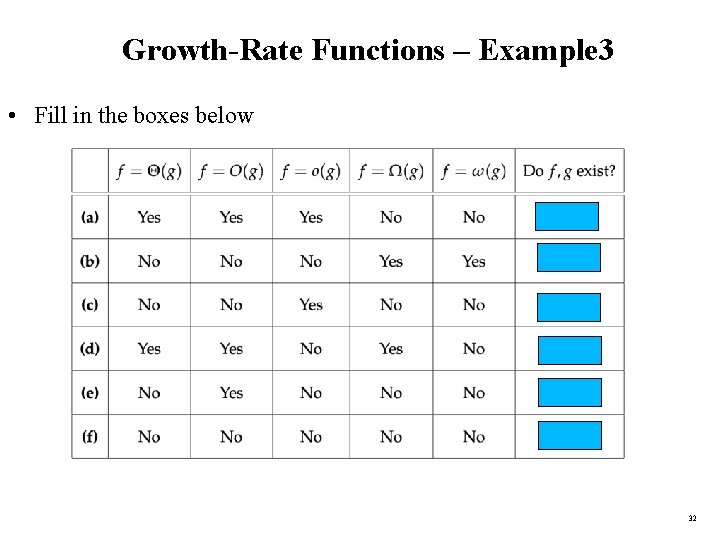Growth-Rate Functions – Example 3 • Fill in the boxes below 32Growth-Rate Functions – Examples 4 -5 • For nxn matrices, time complexity is: O(n 3) • How about this one? O(logn) 33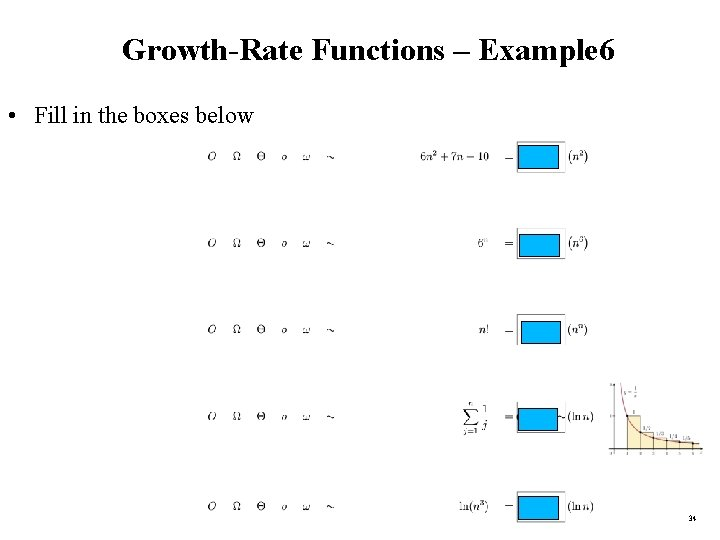Growth-Rate Functions – Example 6 • Fill in the boxes below 34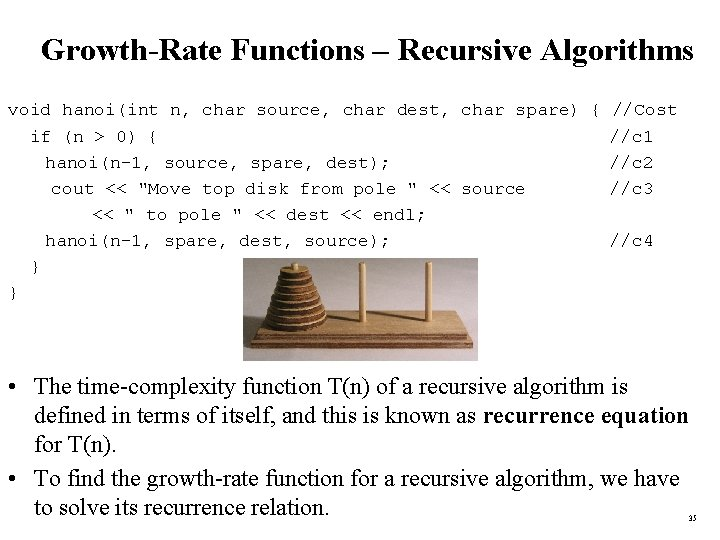Growth-Rate Functions – Recursive Algorithms void hanoi(int n, char source, char dest, char spare) { //Cost if (n > 0) { //c 1 hanoi(n-1, source, spare, dest); //c 2 cout << "Move top disk from pole " << source //c 3 << " to pole " << dest << endl; hanoi(n-1, spare, dest, source); //c 4 } } • The time-complexity function T(n) of a recursive algorithm is defined in terms of itself, and this is known as recurrence equation for T(n). • To find the growth-rate function for a recursive algorithm, we have to solve its recurrence relation. 35Growth-Rate Functions – Hanoi Towers • What is the cost of hanoi(n, ’A’, ’B’, ’C’)? when n=0 T(0) = c 1 when n>0 T(n) = c 1 + c 2 + T(n-1) + c 3 + c 4 + T(n-1) = 2*T(n-1) + (c 1+c 2+c 3+c 4) = 2*T(n-1) + c recurrence equation for the growth-rate function of hanoi-towers algorithm • Now, we have to solve this recurrence equation to find the growth-rate function of hanoi-towers algorithm 36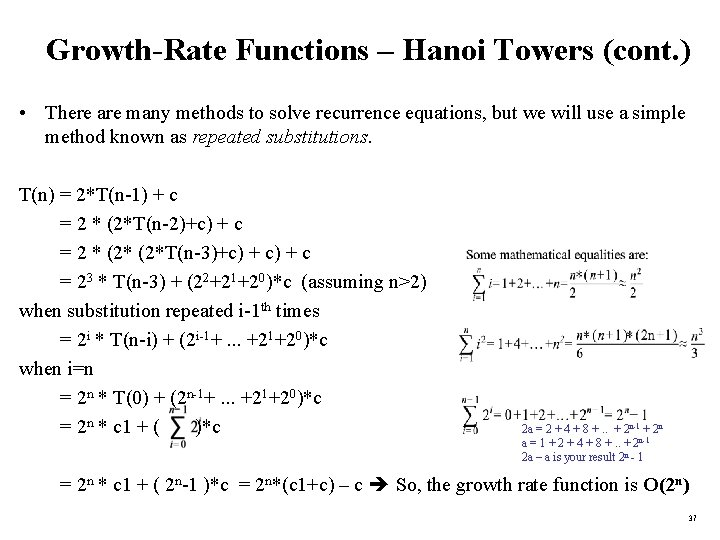Growth-Rate Functions – Hanoi Towers (cont. ) • There are many methods to solve recurrence equations, but we will use a simple method known as repeated substitutions. T(n) = 2*T(n-1) + c = 2 * (2*T(n-2)+c) + c = 2 * (2*T(n-3)+c) + c = 23 * T(n-3) + (22+21+20)*c (assuming n>2) when substitution repeated i-1 th times = 2 i * T(n-i) + (2 i-1+. . . +21+20)*c when i=n = 2 n * T(0) + (2 n-1+. . . +21+20)*c = 2 n * c 1 + ( )*c 2 a = 2 + 4 + 8 +. . + 2 n-1 + 2 n a = 1 + 2 + 4 + 8 +. . + 2 n-1 2 a – a is your result 2 n - 1 = 2 n * c 1 + ( 2 n-1 )*c = 2 n*(c 1+c) – c So, the growth rate function is O(2 n) 37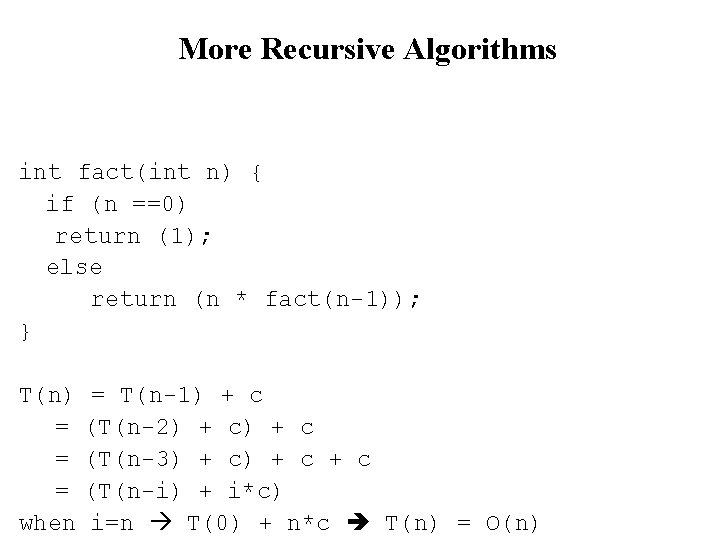More Recursive Algorithms int fact(int n) { if (n ==0) return (1); else return (n * fact(n-1)); } T(n) = T(n-1) + c = (T(n-2) + c = (T(n-3) + c + c = (T(n-i) + i*c) when i=n T(0) + n*c T(n) = O(n)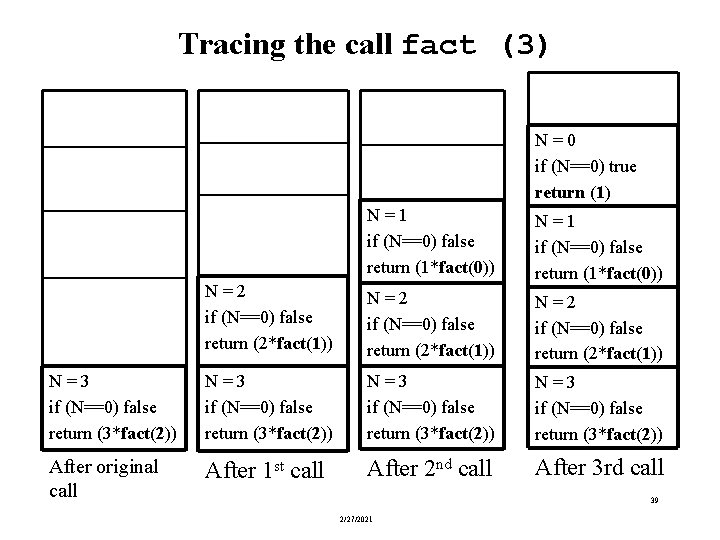Tracing the call fact (3) N = 0 if (N==0) true return (1) N = 1 if (N==0) false return (1*fact(0)) N = 2 if (N==0) false return (2*fact(1)) N = 3 if (N==0) false return (3*fact(2)) After original call After 1 st call After 2 nd call After 3 rd call 39 2/27/2021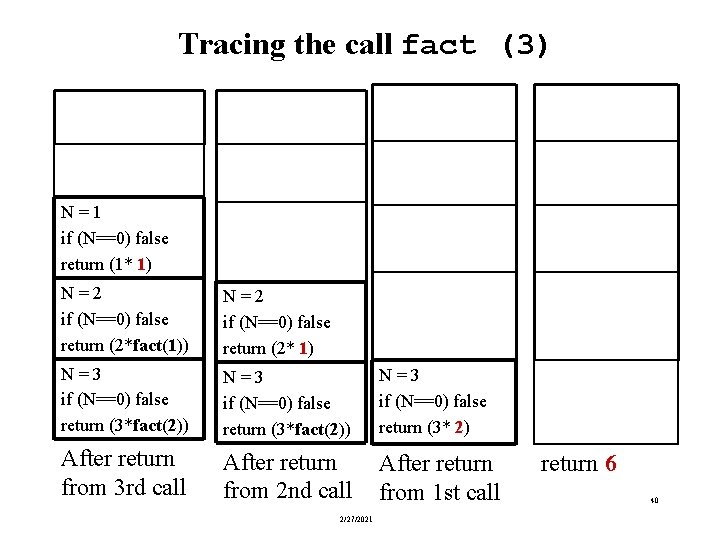Tracing the call fact (3) N = 1 if (N==0) false return (1* 1) N = 2 if (N==0) false return (2*fact(1)) N = 2 if (N==0) false return (2* 1) N = 3 if (N==0) false return (3*fact(2)) N = 3 if (N==0) false return (3* 2) After return from 3 rd call After return from 2 nd call After return from 1 st call 2/27/2021 return 6 40More Recursive Algorithms bool is. Palindrome (string s) { if (s. length() <= 1) return true; return (s==s[s. length()-1]) && is. Palindrome(s. substr(1, s. length()-2)); } T(n) = = = when = T(n-2) + c (T(n-4) + c (T(n-6) + c + c (T(n-i) + i/2*c) i=n T(0) + n/2*c T(n) = O(n)More Recursive Algorithms int fib(int n) { //1 1 2 3 5 8 13 21 34. . }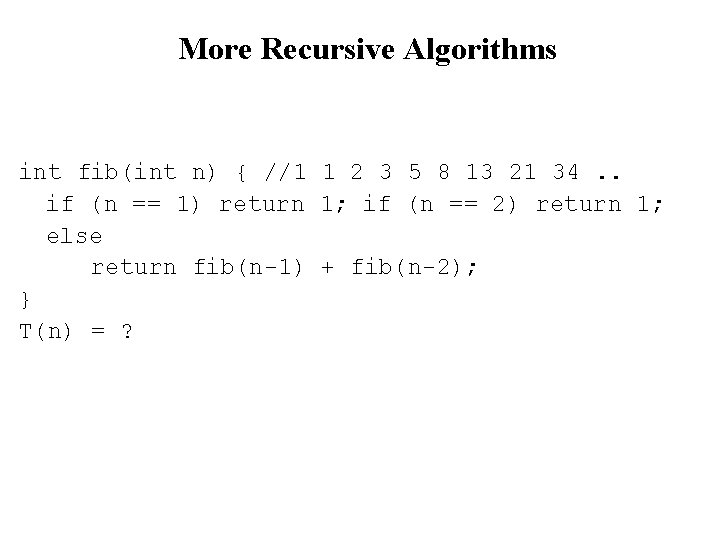More Recursive Algorithms int fib(int n) { //1 1 2 3 5 8 13 21 34. . if (n == 1) return 1; if (n == 2) return 1; else return fib(n-1) + fib(n-2); } T(n) = ?More Recursive Algorithms int fib(int n) { //1 1 2 3 5 8 13 21 34. . if (n == 1) return 1; if (n == 2) return 1; else return fib(n-1) + fib(n-2); } T(n) = T(n-1) + T(n-2) + c = (T(n-2) + T(n-3) + c) + (T(n-3) + T(n-4) + c = gets nasty if we continue substituting; let’s be smarter.More Recursive Algorithms int fib(int n) { //1 1 2 3 5 8 13 21 34. . if (n == 1) return 1; if (n == 2) return 1; else return fib(n-1) + fib(n-2); } T(n) = T(n-1) + T(n-2) + c < T(n-1) + c = O(2 n) as we showed in slide 37. This shows that it cannot exceed O(2 n), hence an upper bound.More Recursive Algorithms int fib(int n) { //1 1 2 3 5 8 13 21 34. . if (n == 1) return 1; if (n == 2) return 1; else return fib(n-1) + fib(n-2); } T(n) = T(n-1) + T(n-2) + c < T(n-1) + c = O(2 n) as we showed in slide 37. This shows that it cannot exceed O(2 n), hence an upper bound. let’s go for the lower bound; if it’s (2 n), then T(n) is (2 n). T(n-1) + T(n-2) + c > T(n-2) + c //cos T(n-1) > T(n-2) slightly. T(n) = 2 T(n-2) + c = 2(2 T(n-4) + c = 4 T(n-4) + 3 c = 2(2(2 T(n-6) + c) + c = 8 T(n-6) + 7 c = = 16 T(n-8) + 15 c = 2 i. T(n-2 i) + (2 i-1) when i = n/2 2 n/2 T(0) + 2 n/2 -1 = (2 n) is the lower bound for T(n).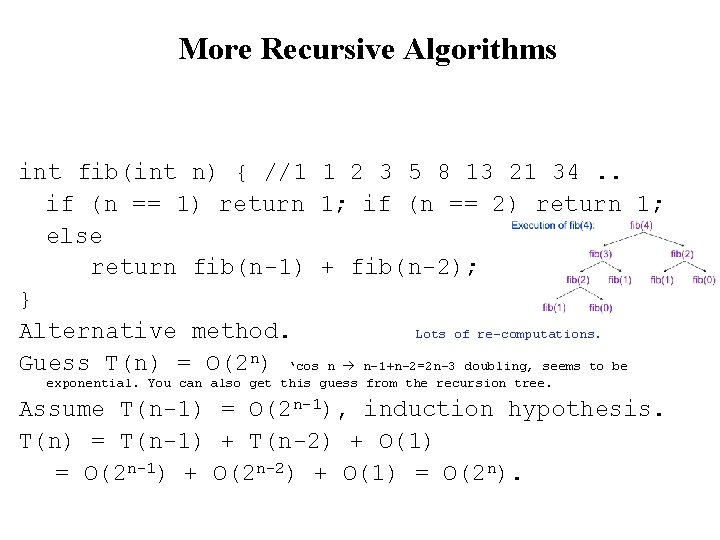More Recursive Algorithms int fib(int n) { //1 1 2 3 5 8 13 21 34. . if (n == 1) return 1; if (n == 2) return 1; else return fib(n-1) + fib(n-2); } Alternative method. Lots of re-computations. Guess T(n) = O(2 n) ‘cos n n-1+n-2=2 n-3 doubling, seems to be exponential. You can also get this guess from the recursion tree. Assume T(n-1) = O(2 n-1), induction hypothesis. T(n) = T(n-1) + T(n-2) + O(1) = O(2 n-1) + O(2 n-2) + O(1) = O(2 n).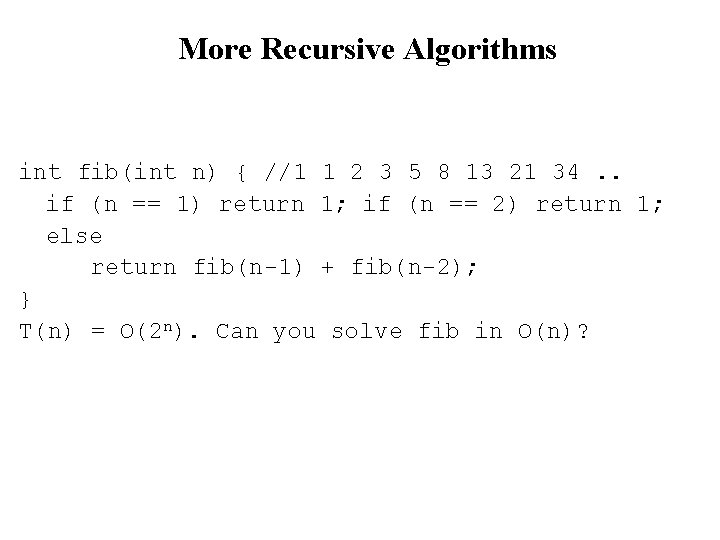More Recursive Algorithms int fib(int n) { //1 1 2 3 5 8 13 21 34. . if (n == 1) return 1; if (n == 2) return 1; else return fib(n-1) + fib(n-2); } T(n) = O(2 n). Can you solve fib in O(n)?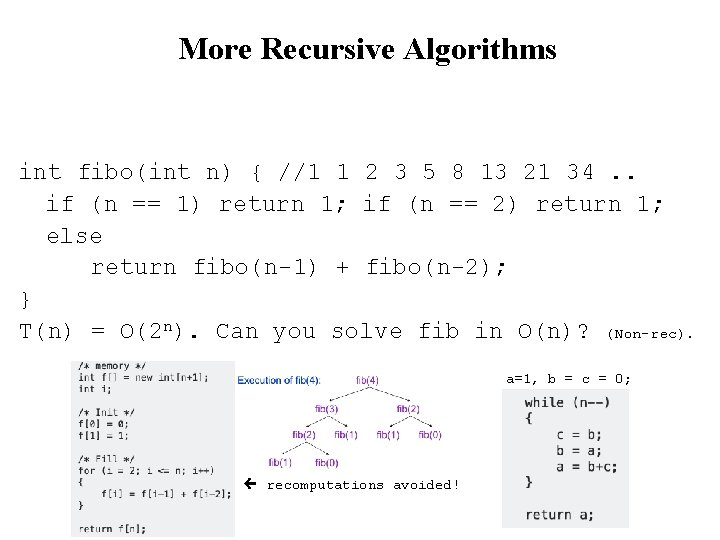More Recursive Algorithms int fibo(int n) { //1 1 2 3 5 8 13 21 34. . if (n == 1) return 1; if (n == 2) return 1; else return fibo(n-1) + fibo(n-2); } T(n) = O(2 n). Can you solve fib in O(n)? (Non-rec). a=1, b = c = 0; or recomputations avoided!More Recursive Algorithms Count positive integers in an array recursively? int count. Pos(int* A, int j) { }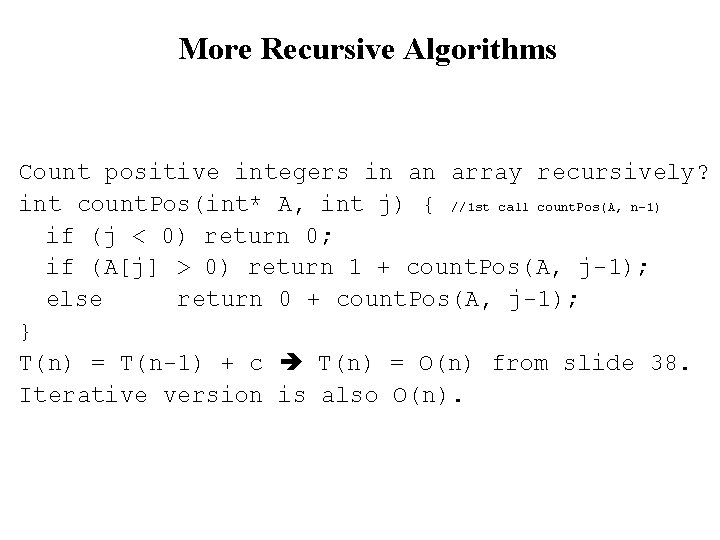More Recursive Algorithms Count positive integers in an array recursively? int count. Pos(int* A, int j) { //1 st call count. Pos(A, n-1) if (j < 0) return 0; if (A[j] > 0) return 1 + count. Pos(A, j-1); else return 0 + count. Pos(A, j-1); } T(n) = T(n-1) + c T(n) = O(n) from slide 38. Iterative version is also O(n).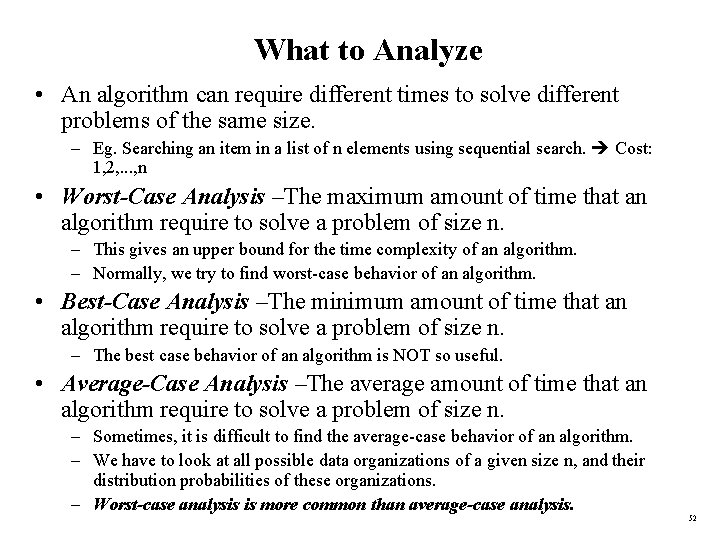What to Analyze • An algorithm can require different times to solve different problems of the same size. – Eg. Searching an item in a list of n elements using sequential search. Cost: 1, 2, . . . , n • Worst-Case Analysis –The maximum amount of time that an algorithm require to solve a problem of size n. – This gives an upper bound for the time complexity of an algorithm. – Normally, we try to find worst-case behavior of an algorithm. • Best-Case Analysis –The minimum amount of time that an algorithm require to solve a problem of size n. – The best case behavior of an algorithm is NOT so useful. • Average-Case Analysis –The average amount of time that an algorithm require to solve a problem of size n. – Sometimes, it is difficult to find the average-case behavior of an algorithm. – We have to look at all possible data organizations of a given size n, and their distribution probabilities of these organizations. – Worst-case analysis is more common than average-case analysis. 52Sequential Search int sequential. Search(const int a[], int item, int n){ for (int i = 0; i < n && a[i]!= item; i++); if (i == n) return – 1; return i; } Unsuccessful Search: O(n) Successful Search: Best-Case: item is in the first location of the array O(1) Worst-Case: item is in the last location of the array O(n) Average-Case: The number of key comparisons 1, 2, . . . , n O(n) 53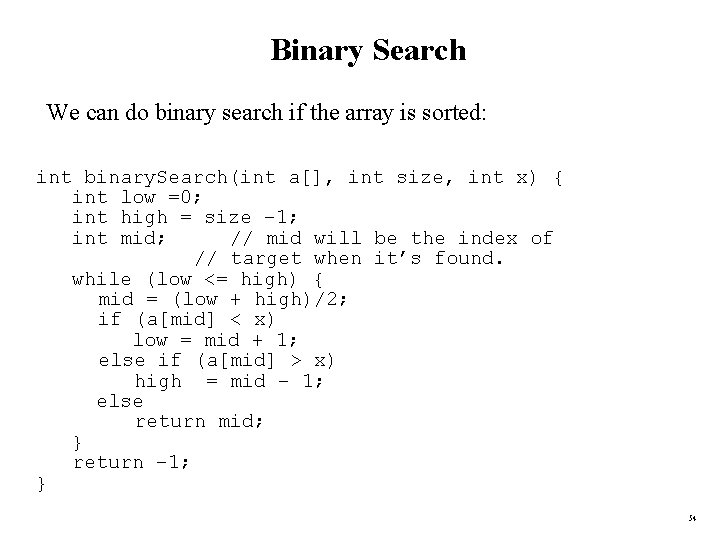Binary Search We can do binary search if the array is sorted: int binary. Search(int a[], int size, int x) { int low =0; int high = size – 1; int mid; // mid will be the index of // target when it’s found. while (low <= high) { mid = (low + high)/2; if (a[mid] < x) low = mid + 1; else if (a[mid] > x) high = mid – 1; else return mid; } return – 1; } 54Binary Search – Analysis • For an unsuccessful search: – The number of iterations in the loop is log 2 n + 1 O(log 2 n) • For a successful search: – Best-Case: The number of iterations is 1. O(1) – Worst-Case: The number of iterations is log 2 n +1 O(log 2 n) – Average-Case: The avg. # of iterations < log 2 n O(log 2 n) 0 3 1 2 2 3 3 1 4 3 5 2 6 3 an array with size 7 # of iterations The average # of iterations = 17/7 = 2. 4285 < log 27 For a proof of the general case see: http: //raider. mountunion. edu/~cindricbb/sp 06/340/avgbinsearch. html 55How much better is O(log 2 n)? n 16 64 256 1024 16, 384 131, 072 262, 144 524, 288 1, 048, 576 1, 073, 741, 824 O(log 2 n) 4 6 8 10 14 17 18 19 20 30 56